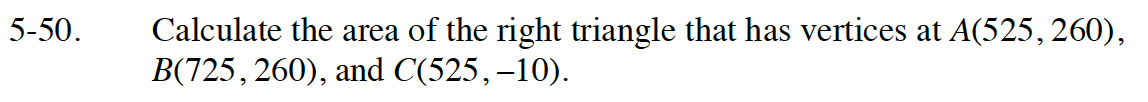Home > CC4 > Chapter 5 > Lesson 5.2.1 > Problem5-50

5-50.

Calculate the area of the right triangle that has vertices at A(525, 260), B(725, 260), and C(525, –10). Homework Help ✎The area of a triangle can be calculated as (0.5)(base · height)
where the base and height must be perpendicular to each other.

Consider graphing the three points and see if you can spot the two sides that are perpendicular.